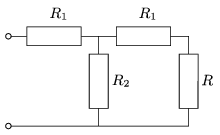Mathematical and Physical Journal
for High Schools
Issued by the MATFUND Foundation
 Already signed up? New to KöMaL?

#Problem P. 4388. (November 2011)

P. 4388. Given the resistances of the resistors R1 and R2. Choose the resistance of the resistor R, such that the equivalent resistance of the circuit shown in the figure,

a) is also R;

b) is a given R0 value. (What values can be given for R0?)(5 pont)

Deadline expired on December 12, 2011.

Sorry, the solution is available only in Hungarian. Google translation

Megoldás. $\displaystyle a)$ $\displaystyle R=\sqrt{R_{1}(R_{1}+2R_{2})}.$

$\displaystyle b)$

$\displaystyle R=\frac{R_{0}(R_{1}+R_{2})-R_{1}(R_{1}+2R_{2})}{R_{1}+R_{2}-R_{0}},$

ahol

$\displaystyle R_{1}+R_{2} \ge R_{0}\ge R_{1}+\frac{R_{1}R_{2}}{R_{1}+R_{2}}.$

Az egyik határeset a matematikailag ,,helytelen'', de fizikailag megvalósítható, reális lehetőségnek, a végtelen $\displaystyle R$-nek (vagyis a szakadásnak) felel meg.

### Statistics:

 155 students sent a solution. 5 points: 57 students. 4 points: 27 students. 3 points: 34 students. 2 points: 25 students. 1 point: 4 students. 0 point: 6 students. Unfair, not evaluated: 2 solutionss.

Problems in Physics of KöMaL, November 2011